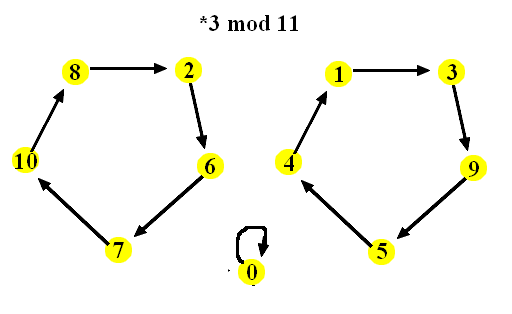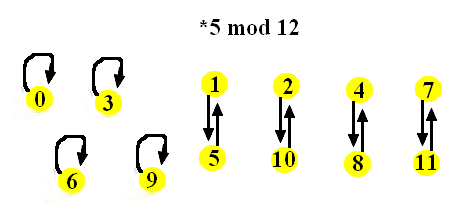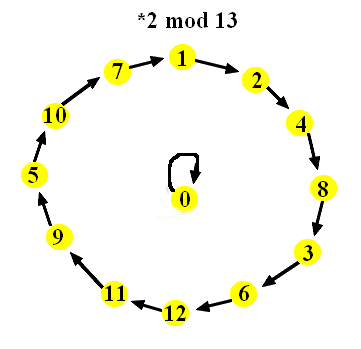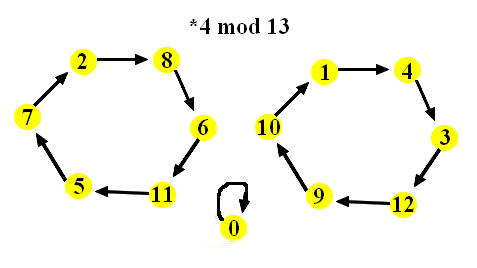# Footnotes on 2.4

## A few more remarks

• Two numbers are equivalent modulo m if their difference is a multiple of m.
• Every integer is equivalent (mod m) to one and only one of the numbers 0, 1, 2, ... n-1. Thus this set 0, 1, ... m-1 represents all integers mod m.
• If a ≡ b (mod m), then a+c ≡ b+c (mod m) and a-c ≡ b-c (mod m) and also a·c ≡ b· c (mod m). Sums, differences, and products where corresponding parts are equivalent modulo m are equivalent modulo m.

## Multiplication by a fixed number modulo mLet us visualize multiplication by 2 modula 11 in the following way: We draw all numbers from 0 to 10 as vertices, similar as for graphs. Remember that every integer is equivalent to some of these numbers modulo 11. Then we draw a directed arc from a to b, a → b, if 2·a ≡ b (mod 11). For instance, 1 → 2, 2 → 4, 4 → 8, 8 → 5 (since 16 ≡ 5 (mod 11)), 5 → 10, 10 → 9, but also 0 → 0. The resutling structure, shown to the right for our example, is called a directed graph or digraph, it is like a graph where all edges are made one-way. Note that at every vertex one arc starts. In our example, one arc also ends at every vertex.

The digraphs for multiplication by 3 or 4 modulo 11 look a little different. But note that even in these two examples one arc ends at every vertex.The digraphs for multiplication by 2, 3, 4, and 5 modulo 12 are shown below. Only in the "*5 mod 12" digraphs at every vertex one arc ends. The other three digraphs have some vertices where more than one arc ends, and some where no arc ends. Why?Below are a few more digraphs. Can you tell in which case we have the property that at every vertex one arc ends?## Solutions of equations modulo m

Is there a solution to the equation 4 · x ≡ 7 (mod 11)? How do we find such a solution? Is there always a solution for the equation
a · x ≡ b (mod 11)
for all numbers a and b? Is there always a solution for the equation
a · x ≡ b (mod 12)
for all numbers a and b? What do these question have to do with what we discussed in the previous section?